# Analysis of Kinematic Processes in Physics Based on Functional-Graphical Lines in Mathematics

Analysis of Kinematic Processes in Physics Based on Functional-Graphical Lines in Mathematics

Zhuldyz NurmaganbetovaNurgali Ashirbayev Manat Shomanbayeva Raina Bekmoldaeva

Department of Mathematics, M. Auezov South Kazakhstan University, 5 Tauke Khan Ave., Shymkent 160012, Republic of Kazakhstan

Department of Informatics, M. Auezov South Kazakhstan University, 5 Tauke Khan Ave., Shymkent 160012, Republic of Kazakhstan

Corresponding Author Email:
zhul.nurmaganbetova@gmail.com
Page:
1503-1507
|
DOI:
https://doi.org/10.18280/mmep.090609
9 September 2022
|
Accepted:
20 November 2022
|
Published:
31 December 2022
| Citation

OPEN ACCESS

Abstract:

An analysis of algebra textbooks of 9 grades allowed to state that in the textbooks little attention is paid to the relationship of the quadratic function with real processes, in some textbooks this connection is completely absent. The purpose of the article is to study the kinematic processes in physics based on functional-graphical lines in mathematics. In order to implement such a connection, we have considered problems with physical content and given methods for solving these problems using a functional graphic line (FGL). The analysis of the given graphs opens up wide methodological possibilities of training, since the graphical representation of the physical process makes it more visual and thereby facilitates understanding of the phenomenon under consideration, promotes the development of abstract thinking, intuition, the ability to analyze and compare, and find a more rational way to solve problems. The systematic implementation of such works allows a deeper study of the topic and rational use of the new technique for studying FGL, finding natural connections between disciplines, studying phenomena and processes in technology and nature from the point of view of functional graphic lines.

Keywords:

function graph, intersubject communication, kinematic process

1. Introduction

School mathematics has a great influence on the intellectual development of students and the ability to learn new information, the flexibility of the mind, criticality, the ability to plan actions, the ability to reason [1-3]. For students to understand the course of school mathematics as a whole, it is important, first of all, that they fully understand the primary concepts. One such concept is function. Functional dependence reflects the practical orientation of the course of mathematics, the relationship of values in the natural sciences, and also forms the functional thinking of students. A significant influence on the content and methodology of teaching function in the school was made by the ideas of mathematics teachers [4-6]. The substantiation of the functional line, as the leading one for the school course in mathematics, is considered one of the major achievements of the mathematics methodology .

As the analysis of the problems of the school course in mathematics has shown, such tasks in the textbooks are not enough. However, it is rather difficult to formulate the concept of the functional dependence of values among schoolchildren. The material studied at the lessons of algebra is formalized in nature, it is not saturated with specific content, which causes difficulties in the understanding of the concept of the functional dependence of quantities among schoolchildren .

An effective condition is to improve the quality of assimilation of the concept of functional dependence in the establishment of intersubject connections between physics and mathematics, which makes it possible to successfully solve contextual problems. In the textbooks, little attention is paid to problems with physical content, as well as tasks that contribute to the formation of such skills that are necessary for learning functions. Problems with physical content use mainly material known to students from a physics course [9-11]. The graphical representation of the physical process makes it more visual and thereby facilitates the understanding of the phenomenon under consideration, contributes to the development of abstract thinking, intuition, the ability to analyze and compare, to find a more rational way to solve problems . In addition, the application of the graphical method helps to strengthen the links between physics and mathematics, fills abstract mathematical laws with concrete physical content [13, 14].

Well-known scientist and methodist A. Ya. Khinchin notes the important role of functional and graphic lines in a school mathematics: “None of the concepts, except functional dependence, can directly and accurately reflect the relationship of motion and real quantities” . The basic concepts in functional – graphic lines are function, function graph, functional dependence. With these concepts, you can display the theoretical problems of the course of mathematics, as well as its practical orientation.

2. Peculiarity of the Motion of a Body Thrown at an Angle to the Horizon

Example 1. In mathematics, when studying the chapter “Elements of trigonometry” , we considered the following example of physical content. “The body is thrown at an angle $\alpha=45^{\circ}$ to the horizon. Show the dependence of the flight range (x) and elevation (h) on the throwing speed of the body (x=f(υ) and h=f(υ)) on one coordinate plane.”

Solution. From the subject “Motion of a body thrown at an angle to the horizon” (Grade 9, ) in physics it is known that (1):

$h=x \cdot \operatorname{tg} \alpha-\frac{g}{2 v_0^2 \cos ^2 \alpha} x^2$                   (1)

Using the conditions of the task, and taking into account that (2):

$h=x-\frac{g}{v_0^2} x^2$                  (2)

when α=450 we fill in the Table 1 for the values $v_0$ (3)

$h=f(x), \frac{x_m}{2}, h_m$                   (3)

Table 1. Values of characteristics $\left(v_0, h, x_m(\mathbf{M}), h_m(\mathbf{M})\right)$ of a thrown body at the angle α=450 to the horizon

 $v_0\left(\frac{m}{s}\right)$ $h=f(x), \alpha=45^0$ $x_m(m)$ $h_m(\boldsymbol{m})$ 10 $x-0.098 x^2$ 10.2 2.55 11 $x-0.081 x^2$ 12.34 3.09 12 $x-0.068 x^2$ 14.7 3.68 14 $x-0.050 x^2$ 20.0 5.0

The dependency graphs $h=f(x)$ are shown in Figure 1, where A(5.1; 2.55); B(6,17; 3.09); C(7.35; 3.68); D(10; 5.0) are the coordinates of the vertices of the parabolas, or the values $\frac{x_m}{2}$ and $h_m$, corresponding to the velocities $v_1, v_2, v_3$ and $v_4$.

## 1.png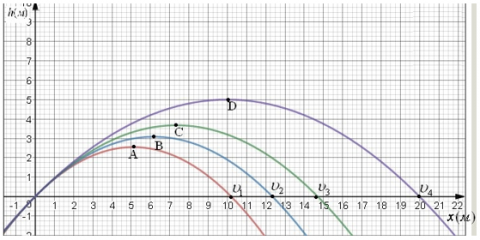Figure 1. Graphs of dependency

Table 2. Values of characteristics $\left(v_0, h, x_m(M), h_m(M)\right)$ of a thrown body at the angle α=300 to the horizon

 $v_0\left(\frac{m}{s}\right)$ $h=f(x), \alpha=30^0$ $x_m(m)$ $h_m(m)$ 10 $0.5774 x-0.06534 x^2$ 8.82 1.28 11 $0.5774 x-0.05299 x^2$ 10.70 1.54 12 $0.5774 x-0.04537 x^2$ 12.74 1.84 14 $0.5774 x-0.03384 x^2$ 17.32 2.50

Now, in order to study the correspondence of movements, we draw the same graphs for α=300 and α=600. The data values are shown in Tables 2 and 3, and comparison graphs are shown in Figure 2.

Table 3. Values of characteristics $\left(v_0, h, x_m(M), h_m(M)\right)$ of a thrown body at the angle α=600 to the horizon

 $v_0\left(\frac{m}{s}\right)$ $h=f(x), \alpha=60^0$ $x_m(m)$ $h_m(m)$ 10 $1.732 x-0.196 x^2$ 8.82 3.83 11 $1.732 x-0.162 x^2$ 10.70 4.63 12 $1.732 x-0.136 x^2$ 12.74 5.51 14 $1.732 x-0.100 x^2$ 17.32 7.50

For: а) α=300, A_1 (4.42; 1.28); B_1 (5.35; 1.54); C_1 (6.37; 1.84); D_1 (8.66; 2.50);

b) α=450, A(5.1; 2.55); B(6.17; 3.09); C(7.35; 3.68); D(10; 5.0);

c) α=600, $A_2(4.42 ; 3.83) ; B_2(5.35 ; 4.63) ; C_2(6.37 ; 5.51) ; D_2(8.66 ; 7.50);$

## 2.png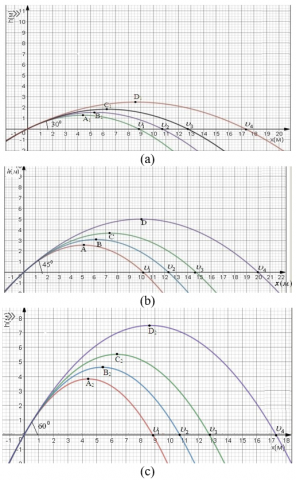Figure 2. a) dependence graph h=f(x) for α=450; b) dependence graph h=f(x) for α=300; c) dependence graph h=f(x) for α=600

Based on a comparison of the graphs in the figures, the following conclusions can be drawn: The height of the lift of the thrown body (h) and the range of flight (x) increase in proportion to the speed of the throw. The maximum flight range is achieved when inclination α=450, and when the throw angles $\left(45^{\circ}+\alpha\right)$ and $\left(45^0-\alpha\right)$, then the flight range of the bodies is the same $\left(0^0 \leq \alpha \leq 45^0\right)$.

3. The Dependence of the Lift Height and the Flight Range of a Body from the Throw Angle

Example 2. Now we will analyze the dependence of the lift height (h) and the flight range (x) of a body thrown at an angle to the horizon at a speed $v_0=10 \frac{\mathrm{m}}{\mathrm{s}}$ from the throw angle (α), plotting their graphs (x=f(α) and h=f(α)) on the same coordinate plane. We use the formula (1) again. Fill in the Table 4 to determine the necessary values for plotting.

Table 4. Values of characteristics $\left(h, x_m(\mathrm{M}), h_m(\mathrm{M})\right)$ of a thrown body at an angle to the horizon with speed $v_0=10 \frac{\mathrm{M}}{\mathrm{c}}$

 α(grad) h=f(x) $\boldsymbol{x}_m(\boldsymbol{m})$ $\boldsymbol{h}_m(\boldsymbol{m})$ 15 $0.268 x-0.053 x^2$ 2.55 0.34 30 $0.577 x-0.065 x^2$ 4.41 1.28 45 $x-0.098 x^2$ 5.10 2.55 60 $1.732 x-0.196 x^2$ 4.41 3.83 75 $3.732 x-0.732 x^2$ 2.55 4.76

The dependency graph h=f(x) is shown in Figure 3. A(2.55; 0.34); B(4.41; 1.28); C(5.10; 2.55); D(4.41; 3.83); E(2.55; 4.76) are the vertices of the parabolas.

## 3.png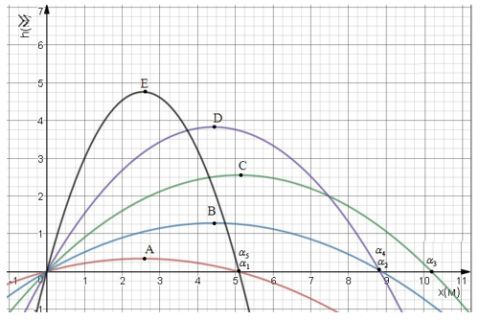Figure 3. Dependence graph $h=f(x)$ at an angle to the horizon with speed $v_0=10 \frac{\mathrm{M}}{\mathrm{c}}$

Conclusion: when the angle of graph inclination $0^0 \leq \alpha \leq 45^0$, the flight range (x) increases in proportion to the angle, while $45^0 \leq \alpha \leq 90^0$ it decreases to zero. Lifting height (h) increases in proportion to the angle of inclination (α). When α=900 the lifting height is determined by the formula $h=\frac{v_0^2}{2 q}$.

4. The Features of the Motion of a Body Thrown Horizontally from a Heigh

Example 3. Consider the motion of a body thrown horizontally from a height $h_0=5 m$ and determine the dependence of the flight range (x) on the speed (υ0) of the throw (x=f(x)). Solution: consider the equation of motion of the body in the form (4)

$h=h_0-\frac{g}{2 v_0^2} \cdot x^2$                  (4)

The values required for building the graphs are shown in Table 5 .

Table 5. Values of characteristic (x, υ0) of a thrown body from a height $h_0=5 M$ horizontally

 $v_0\left(\frac{m}{s}\right)$ $\boldsymbol{h}=\boldsymbol{f}(\boldsymbol{x})$ $\boldsymbol{x}_{\max }$ $h_0(m)$ 3 $5-0.545 x^2$ 3.03 5 5 $5-0.196 x^2$ 5.05 5 7 $5-0.100 x^2$ 7.07 5 8 $5-0.077 x^2$ 8.08 5 10 $5-0.049 x^2$ 10.10 5

Figure 4 shows a dependency graph $h=f\left(v_0\right)$. Conclusion: the flight range of the body (x) increases in proportion to the speed of the throw (υ0), and its trajectory is a branch of the parabola.

## 4.png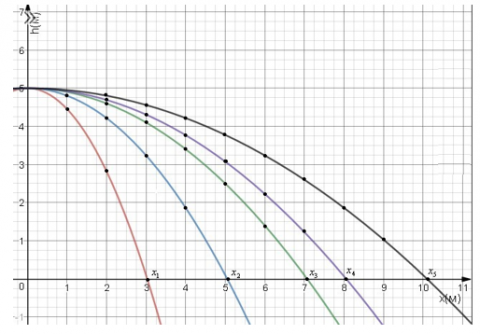Figure 4. Dependence graph $h=f\left(v_0\right)$ of a thrown from a height $h_0=5 M$

Example 4. We graphically study the dependence (5) related to the quadratic function in mathematics, which determines the coordinates of the uniform translational motion in kinematics.

$x=x_0+v_0 t+\frac{a t^2}{2}$                   (5)

Table 6. Values of quantities included in the dependence $x=x_0+v_0 t+\frac{a t^2}{2}$

 $x=f\left(x_0, v_0, \alpha\right)$ 0 0 $a_x>0$ $x=0.5 a t^2$ 0 0 $a_x<0$ $x=-0.5 a t^2$ 0 $v_{0 x}>0$ $a_x>0$ $x=v_0 t+0.5 a t^2$ 0 $v_{0 x}>0$ $a_x<0$ $x=v_0 t-0.5 a t^2$ 0 $v_{0 x}<0$ $a_x>0$ $x=-v_0 t+0.5 a t^2$ 0 $v_{0 x}<0$ $a_x<0$ $x=-v_0 t-0.5 a t^2$ $x_0>0$ $v_{0 x}>0$ $a_x>0$ $x=x_0+v_0 t+0.5 a t^2$ $x_0>0$ $v_{0 x}>0$ $a_x<0$ $x=x_0+v_0 t-0.5 a t^2$ $x_0>0$ $v_{0 x}<0$ $a_x>0$ $x=x_0-v_0 t+0.5 a t^2$ $x_0>0$ $v_{0 x}<0$ $a_x<0$ $x=x_0-v_0 t-0.5 a t^2$ $x_0<0$ $v_{0 x}>0$ $a_x>0$ $x=-x_0+v_0 t+0.5 a t^2$ $x_0<0$ $v_{0 x}>0$ $a_x<0$ $x=-x_0+v_0 t-0.5 a t^2$ $x_0<0$ $v_{0 x}<0$ $a_x>0$ $x=-x_0+v_0 t+0.5 a t^2$ $x_0<0$ $v_{0 x}<0$ $a_x<0$ $x=-x_0+v_0 t-0.5 a t^2$

The graphs of these functions depend on the number of quantities $x_0, v_0$ and $a$ which entering in it and their directions. In this regard, we present possible situations that will take these values depending on the type of processes (Table 6). Here: $v_{0 x}$ and $a_x$ are the projections of the velocity and acceleration of the body on the axis (x) .

When using values $\left|x_0\right|=2 \mathrm{M} ;\left|v_0\right|=2 \frac{\mathrm{M}}{\mathrm{c}} ;|a|=2 \frac{\mathrm{M}}{\mathrm{c}^2}$ on the interval 0≤t≤4, the graphs of these dependencies are compressed. Dependency graphs are shown in Figures 5 and 6.

## 5.png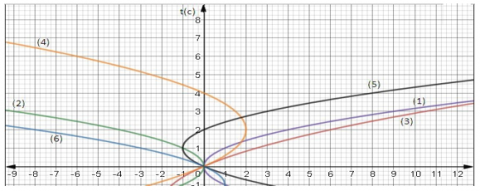Figure 5. Graphs of the dependence of a quadratic function in mathematics (1-6)

## 6.png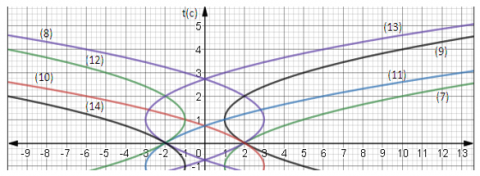Figure 6. Graphs of the dependence of a quadratic function in mathematics (7-14)

Based on the built-in graphs, the following conclusions can be drawn:

1. All graphs are parabolic curves, and in six cases $\left(x_4, x_5, x_8, x_9, x_{12}, x_{13}\right)$ you can find the vertices of parabolas.

2. The graphs are symmetrical with each other in comparison with the axes: $\left(x_3\right.$ and $x_6 ; x_1$ and $x_2 ; \,x_4$ and $x_5 ; \, x_7$ and $x_{14} ; \, x_8$ and $x_{13} ; \, x_9$ and $x_{12} ; \,x_{11}$ and $x_{10}$ ).

Note. Symmetry is performed when the signs of the corresponding dependency members are mutually opposite. We will verify that $x_{10}$ and $x_{11}$ are indeed symmetric (6, 7, 8).

$x_{10}=x_0-v_0 t-0.5 a t^2$                        (6)

$x_{11}=-x_0-v_0 t-0.5 a t^2$                        (7)

$x_{10}=x_0-v_0 t-0.5 a t^2=-\left(-x_0+v_0 t+0.5 a t^2\right)=-x_{11}$                  (8)

3. When the signs of the corresponding dependency members are the same, the body moves in one direction. For example, $x_3 ; x_5 ; x_7$; etc.

If the signs of the terms are different, then the body will move in two directions along the selected axis. For example, $x_4 ; x_6 ; x_{12}$; etc. Another type of translational motion in kinematics is a smooth rectilinear motion. Here, to study the motion, the equation of motion (x=f(x)) and the velocity equation (v=f(t)) are used. The general form of the equations is written as follows: $x=x_0+v_0 t$ and $v=v_0+a t$. Both equations relate to the linear equation (y=kx+b), which is studied in mathematics. Here: y and x  variables, k≠0, b are constant numbers. In our case: x , v and t are the variables; $x_0, v_0, v_0 \neq 0, a \neq 0$ are the constant values, namely: x0 is an initial coordinate; $v_{0 x}$ is a starting speed; a is an acceleration. Now, for each equation, we analyze the motion of the body. Tables 7 and 8 give a range of values of the quantities included in the equation that they can take in the general case. And their graphs are shown in Figures 7 and 8. Here: $x_0=4 m; \, v_0=3 \frac{m}{s} ;$ and $v_0=4 \frac{m}{s} ; \, a=2 \frac{m}{s^2}$.

Table 7. Range of values of quantities included in the equation $x=x_0+v_0 t$

 $x_0(m)$ $v_0\left(\frac{m}{s}\right)$ $x=f(t)$ 0 $v_{0 x}>0$ $x=v_0 t$ 0 $v_{0 x}<0$ $x=-v_0 t$ $x_0>0$ $v_{0 x}>0$ $x=x_0+v_0 t$ $x_0>0$ $v_{0 x}<0$ $x=x_0-v_0 t$ $x_0<0$ $v_{0 x}>0$ $x=-x_0+v_0 t$ $x_0>0$ $v_{0 x}<0$ $x=-x_0-v_0 t$ $x_0>0$ 0 $x=x_0$ $x_0<0$ 0 $x=-x_0$

Table 8. Range of values of quantities included in the equation $v=v_0+a t$

 $v_0\left(\frac{m}{s}\right)$ $a\left(\frac{m}{s^2}\right)$ $v=f(t)$ 0 $a_x>0$ $v=a t$ 0 $a_x<0$ $v=-a t$ $v_{0 x}>0$ $a_x>0$ $v=v_0+a t$ $v_{0 x}>0$ $a_x<0$ $v=v_0-a t$ $v_{0 x}<0$ $a_x>0$ $v=-v_0+a t$ $v_{0 x}<0$ $a_x<0$ $v=-v_0-a t$ $v_{0 x}>0$ 0 $v=v_0$ $v_{0 x}<0$ 0 $v=-v_0$

## 7.pngFigure 7. Graphs of linear function $x=x_0+v_0 t$

## 8.png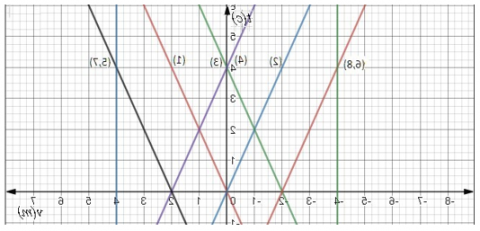Figure 8. Linear function $v=v_0+a t \text { graphs }$

As a result of the analysis of the graphs shown in Figures 7 and 8, the following conclusions can be drawn :

1. Due to the fact that both dependencies x=f(t) and υ=f(t) are defined by linear functions, in accordance with the terms of the graphics are similar.

2. Graphs are straight lines, since $v_0$ and $a$ are independent of the projection on the coordinate axis.

3. And depending on the sign of the projections within the coordinates [20, 21]:

- is in the I quarter, when the signs are positive;

- located in the II quarter, when the signs are negative;

- when the signs of the members are both positive and negative, they are in both quarters. For example: $x_4$ and $x_5 ; \,v_4$ and $v_5$.

5. Conclusions

The use of interdisciplinary relations allows, on the other hand, to fulfill the requirements set in the curriculum. We examined the possibilities of studying kinematic processes in physics while studying functional graphical lines in mathematics, where the functional relationships between quantities can be shown to students in terms of dynamic processes using a graph. As a rule, to determine the position (coordinates) of a body uniformly moving in a straight line, we use a graph of a linear function to draw a straight line on the coordinate plane, and for a body in smooth translational motion, we draw a parabola on a plane, but this does not allow fully take into account the conditions of the problem of finding the coordinates of a moving body; a drawn graph can give an explanation of only one case.

Thus, all the above materials lead to the following conclusions:

1. When studying various functions in mathematics on the basis of a graphical analysis of the kinematic processes of physics, it is possible to widely and systematically use the possibilities of the correct assimilation of functional-graphic lines in students.

2. The systematic conduct of such work allows a deeper study of the topic, to find natural connections between disciplines, studying phenomena and processes in technology and nature from the point of view of functional graphic lines.

3. It is necessary to develop the ability of students to be critical of graphic images and should encourage the image of various laws using mathematical graphs, including using a computer.

References

 Dmytruk, A.A., Gatalevych, A.I., Kuchma, M.I. (2022). Stable range conditions for abelian and duo rings. Matematychni Studii, 57(2): 92-97.

 Dzhomartova, S.A., Mazakov, T.Z., Karymsakova, N.T., Zhaydarova, A.M. (2014). Comparison of two interval arithmetic. Applied Mathematical Sciences, 8(72): 3593-3598. http://dx.doi.org/10.12988/ams.2014.44301

 Mukhambetzhanov, S.T., Baishemirov, Z.D. (2013). Procedure of evaluation development for drilling-in and well completion. World Applied Sciences Journal, 24(2): 168-174.

 Berikhanova, G. E., Zholymbaev, O. M., Mussatayeva, I. S. (2017). On some integral inequalities for convex functions. Far East Journal of Mathematical Sciences, 101(12): 2645-2651.

 Mordkovich, A.G. (2012). About some problems of school mathematical education. Mathematics in School, 10: 35-43.

 Popad'ina, S.Y. (2009). Implementation of the functional-graphic line in personalized teaching of a general mathematics course using the MATHCAD computer system. http://netess.ru/3pedagogika/504691-1-realizaciya-funkcionalno-graficheskoy-linii-personalizirovannom-obuchenii-obscheobrazovatelnomu-kursu-matematiki-ispolzovani.php, accessed on Sep. 17, 2022.

 Tang, J.G., Mukhambetzhanov, S., Nulahemaiti, B., Baishemirov, Z. (2014). Method of an evaluation of formation exposing with preliminary data. Life Science Journal, 11(10s): 321-326.

 Mustafin, A.T., Volkov, E.I. (1984). The role of lipid and antioxidant exchanges in cell division synchronization (mathematical model). Biological Cybernetics, 49(3): 149-154. https://doi.org/10.1007/BF00334460

 Sarantsev, G.I. (2001). Methodology of teaching mathematics. Krasniy Oktyabr, Saransk.

 Fridman, L.M. (2005). Theoretical foundations of teaching mathematics. LLC Rokhos, Moscow.

 Tikhonova, L.V. (2002). Methodological features of the formation of a functionally graphic line of the algebra course in the conditions of personality-oriented learning. http://www.dslib.net/teoria-vospitania/metodicheskie-osobennosti-formirovanija-funkcionalno-graficheskoj-linii-kursa.html, accessed on Sep. 28, 2022.

 Antonova, I.V. (2003). Differentiated work of a teacher of mathematics in the formation of the concept of function in the course of algebra of a primary school. https://www.dissercat.com/content/differentsirovannaya-rabota-uchitelya-matematiki-pri-formirovanii-ponyatiya-funktsii-v-kurse, accessed on Sep. 14, 2022.

 Antonova, I.V., Uteeva, R.A. (2002). Level differentiation in the study of the concept of function in the 7th grade. Developing the potential of mathematics and its implementation in learning. Arzamas State Pedagogical Institute, Arzamas.

 Mishenina, O.V. (2004). Theory and methods of studying functions in a basic school in the context of modular learning. https://www.dissercat.com/content/teoriya-i-metodika-izucheniya-funktsii-v-osnovnoi-shkole-v-kontekste-modulnogo-obucheniya, accessed on Sep. 13, 2022.

 Abramova, O.V. (2012). Formation in students of the basic school of skills to work with function graphs in the context of the implementation of intersubject connections in physics, mathematics and computer science. Moscow International University, Moscow.

 Mustafin, A. (2006). Two mutually loss-coupled lasers featuring astable multivibrator. Physica D: Nonlinear Phenomena, 218(2): 167-176. https://doi.org/10.1016/j.physd.2006.05.003

 Taschner, R. (2013). Number coming from cold. When math becomes adventure. Azbuka-Atticus, Moscow.

 Abylkasymova, A. (2019). Algebra. Almaty: Mektep.

 Kazakbaeva, D.M., Nasokhova, Sh.B., Bekbasar, N. (2019). Physics. Mektep, Almaty.

 Al-Habahbeh, O.M., Al-Adwan, R.S. (2022). Dynamic modelling of docking autonomous PODs in tandem configuration. Mathematical Modelling of Engineering Problems, 9(4): 955-963. https://doi.org/10.18280/mmep.090412

 AlKhulaifi, D., AlQahtani, M., AlSadeq, Z., Rahman, A. Musleh, D. (2022). An overview of self-adaptive differential evolution algorithms with mutation strategy. Mathematical Modelling of Engineering Problems, 9(4): 1017-1024. https://doi.org/10.18280/mmep.090419